# How To Test Autoclave Heating Elements

Don't Want To Do The Math?

We make it simple.  To see what the test results for the heater on your autoclave should be, just find the heating element for your autoclave here on this site.  The ranges for your model are given right after the description

The fact that a heating element shows continuity does not tell you whether the heating element is good, only that there are no breaks in the wiring. There could still be a short somewhere within the element itself causing your heating problems and, with our guide below, checking it is a very simple procedure.

Testing The Heating Elements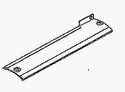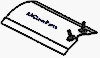This test consists of measuring the amount of resistance in the element. To do this, first, turn the autoclave off and unplug it from the wall

WARNING: CURRENT MUST NOT BE PRESENT WHEN MEASURING RESISTANCE.

Next, determine what the resistance value is. The heating elements located here on our site show the resistance values, but we have included the formulas below if you need, or want, to calculate them on your own.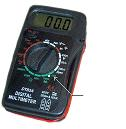Now set your volt/ohm meter on 200 ohms (200 NOT 200k). The ohms range is located within the Omega ( Ω )(look for this symbol)  section of your meter.   It is also the area with "k" ranges (ie:  2000k,. 200k, 20K and then 2000, 200).

Place a probe from the volt/ohm meter on each wire where it connects to the element (it really doesn't matter which color probe goes where). Write the readings from your meter down. Be sure to test all of the elements and compare your readings to the ranges for your heating element as determined below.

For the heating element to be good, it must fall within a range of (+) or (-) 10%. For example, if the heating element you are testing has a resistance value of 9 ohms, the range is 8.1 to 9.9.

This range is determined by subtracting and adding 10% to the rating for your element, in this case, the rating is 9 ohms.  So you would subtract  10% (0.9) from 9.00 and then add 10% (0.9) to 9.00 (Thus 8.1 to 9.9).   If the element you are testing falls outside of the range for your element, then it is bad and replacement is required.

Your Done!  (See, we told you it was simple!)

Simpler Yet, Forget The Math!  As instructed above, just find the heating element for your autoclave here on our site.  The Ranges Are Given - No Math Required!

Electrical Formulas Needed For Testing The Heating Elements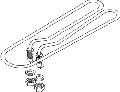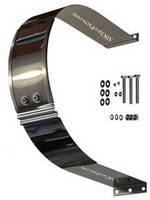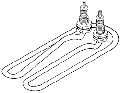Symbols:

V=Volts
A= Amps (Current)
W=Watts
R = Resistance (measured in OHMS)

To find the number of Volts, the formula is:

• V A) x (R)
(Volts is equal to the number of Amps multiplied by the number of Resistance (ohms)

Example: A Heating Element Pulling 13.333 Amps with a Resistance Level of 9.000 OHMS

V13.333) x (9.000) = 120 Volts (rounded Off). So the heating element is for a 120 Volt unit

To find the number of Amps, the formula is:

• A W) / (V)
(Amps is equal to Watts divided by Volts)

Example: A 1600 Watt, 120 Volt Heating Element

A 1600) / (120) = 13.333 Amps. So the heating element is Pulling 13.333 Amps

To find the Resistance, the formula is

• R V) / (A)
(Resistance is equal to the number of Volts divided by the number of Amps)

Example: A 120 Volt Heating Element is Pulling 13.333 Amps

R 120) / (13.333) R = 9 Ohms (Resistance is measured in OHMS) So the heating element has a resistance value of 9.000 OHMS

To find the number of Watts, the formula is

• W V) x (A)
(Watts is equal to the Number of Volts multiplied by the number of Amps)

Example: A 120 volt heating element is Pulling 13.333 amps

W 120) x (13.333) W = 1600 Watts (Rounded Off)